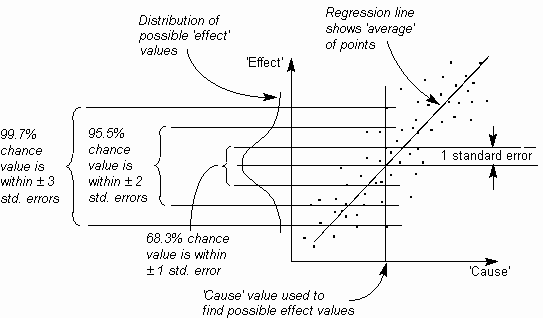The Psychology of Quality and More

# Regression line

In drawing a Scatter Diagram, there are a number of points which form a 'rough' line. It can be useful to plot a solid line along the 'center-line' of these points, typically so you can use the graph for some kind of estimation, or to compare it with a previous plot.

The regression line is commonly calculated with 'the method of least squares'.Correlation, Correlation coefficient, Correlation Table, Scatter Diagram

### You can buy books here

And the big
paperback book